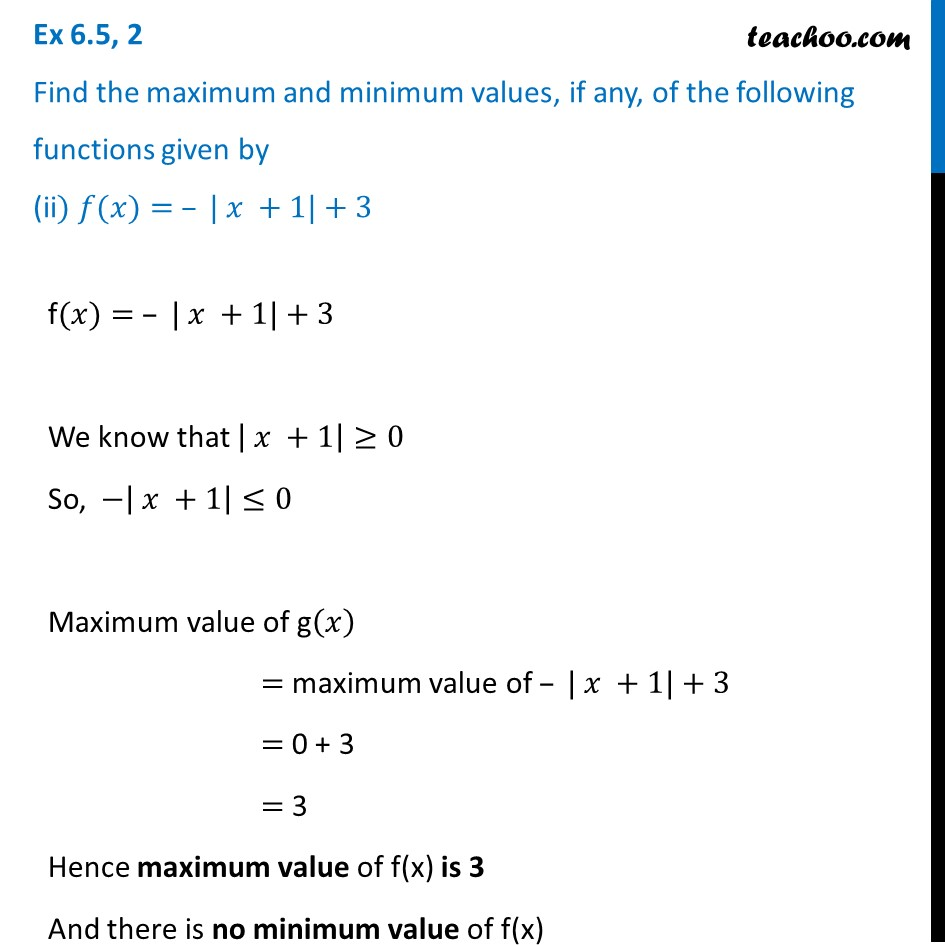Ex 6.5

Chapter 6 Class 12 Application of Derivatives
Serial order wiseMaths Crash Course - Live lectures + all videos + Real time Doubt solving!

### Transcript

Ex 6.5, 2 Find the maximum and minimum values, if any, of the following functions given by (ii) 𝑓(𝑥)= – | 𝑥 +1|+3f(𝑥)= – | 𝑥 +1|+3 We know that | 𝑥 +1|≥0 So, −| 𝑥 +1|≤0 Maximum value of g(𝑥) = maximum value of – | 𝑥 +1|+3 = 0 + 3 = 3 Hence maximum value of f(x) is 3 And there is no minimum value of f(x)